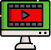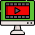VideosNotes

### Approximation

Quadratic Equation
A quadratic equation is an equation of the second degree, meaning it contains at least one term that is squared. The standard form is ax² + bx + c = 0 with a, b, and c being constants, or numerical coefficients, and x is an unknown variable.
Views: N/A
Video Duration: 9:23
Date Uploaded: November 03, 2020
Grade Level: SS 2
Share this with your friends!
On the Same TopicQuadratic Equations.
A quadratic equation is an ...
Other Related Videos